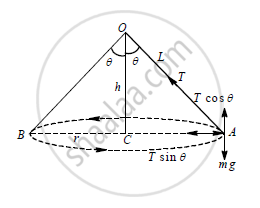Share

# A Stone of Mass 1 kg is Whirled in Horizontal Circle Attached at the End of a 1 m Long String. If the String Makes an Angle of 30º with Vertical, Calculate the Centripetal Force Acting on the Stone. - Physics

#### Question

A stone of mass 1 kg is whirled in horizontal circle attached at the end of a 1 m long string. If the string makes an angle of 30º with vertical, calculate the centripetal force acting on the stone.(g=9.8m/s2).

#### Solutionm = 1kg

Tcos30^@= mg

T=(mg)/(cos30^@)

F_c = Tsin30^@

F_c=(mg)/cos30^@""xxsin30^@

=mg × tan30º

= 1 × 9.8 × 1/√3

= 9.8/1.732

=5.658N

the centripetal force acting on the stone is 5.658N

Is there an error in this question or solution?